Intermediate Algebra 2e

# 3.2Slope of a Line

Intermediate Algebra 2e3.2 Slope of a Line

### Learning Objectives

By the end of this section, you will be able to:

• Find the slope of a line
• Graph a line given a point and the slope
• Graph a line using its slope and intercept
• Choose the most convenient method to graph a line
• Graph and interpret applications of slope–intercept
• Use slopes to identify parallel and perpendicular lines

### Be Prepared 3.4

Before you get started, take this readiness quiz.

Simplify: $(1–4)(8−2).(1–4)(8−2).$
If you missed this problem, review Example 1.30.

### Be Prepared 3.5

Divide: $04,40.04,40.$
If you missed this problem, review Example 1.49.

### Be Prepared 3.6

Simplify: $15−3,−153,−15−3.15−3,−153,−15−3.$
If you missed this problem, review Example 1.30.

### Find the Slope of a Line

When you graph linear equations, you may notice that some lines tilt up as they go from left to right and some lines tilt down. Some lines are very steep and some lines are flatter.

In mathematics, the measure of the steepness of a line is called the slope of the line.

The concept of slope has many applications in the real world. In construction the pitch of a roof, the slant of the plumbing pipes, and the steepness of the stairs are all applications of slope. and as you ski or jog down a hill, you definitely experience slope.

We can assign a numerical value to the slope of a line by finding the ratio of the rise and run. The rise is the amount the vertical distance changes while the run measures the horizontal change, as shown in this illustration. Slope is a rate of change. See Figure 3.5.

Figure 3.5

### Slope of a Line

The slope of a line is $m=riserun.m=riserun.$

The rise measures the vertical change and the run measures the horizontal change.

To find the slope of a line, we locate two points on the line whose coordinates are integers. Then we sketch a right triangle where the two points are vertices and one side is horizontal and one side is vertical.

To find the slope of the line, we measure the distance along the vertical and horizontal sides of the triangle. The vertical distance is called the rise and the horizontal distance is called the run,

### How To

#### Find the slope of a line from its graph using $m=riserun.m=riserun.$

1. Step 1. Locate two points on the line whose coordinates are integers.
2. Step 2. Starting with one point, sketch a right triangle, going from the first point to the second point.
3. Step 3. Count the rise and the run on the legs of the triangle.
4. Step 4. Take the ratio of rise to run to find the slope: $m=riserun.m=riserun.$

### Example 3.12

Find the slope of the line shown.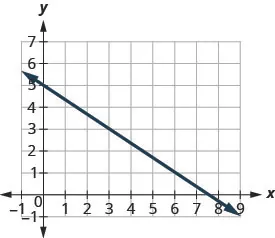### Try It 3.23

Find the slope of the line shown.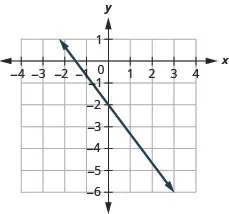### Try It 3.24

Find the slope of the line shown.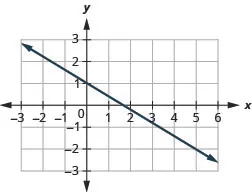How do we find the slope of horizontal and vertical lines? To find the slope of the horizontal line, $y=4,y=4,$ we could graph the line, find two points on it, and count the rise and the run. Let’s see what happens when we do this, as shown in the graph below.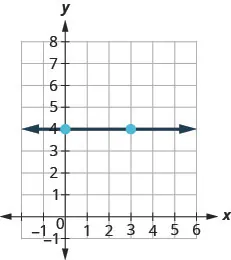What is the rise? The rise is 0. What is the run? The run is 3. What is the slope? $m=riserunm=riserun$ $m=03m=03$ $m=0m=0$ The slope of the horizontal line $y=4is 0.y=4is 0.$

Let’s also consider a vertical line, the line $x=3,x=3,$ as shown in the graph.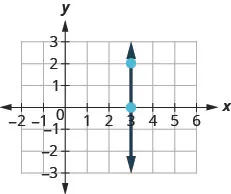What is the rise? The rise is 2. What is the run? The run is 0. What is the slope? $m=riserunm=riserun$ $m=20m=20$

The slope is undefined since division by zero is undefined. So we say that the slope of the vertical line $x=3x=3$ is undefined.

All horizontal lines have slope 0. When the y-coordinates are the same, the rise is 0.

The slope of any vertical line is undefined. When the x-coordinates of a line are all the same, the run is 0.

### Slope of a Horizontal and Vertical Line

The slope of a horizontal line, $y=b,y=b,$ is 0.

The slope of a vertical line, $x=a,x=a,$ is undefined.

### Example 3.13

Find the slope of each line: $x=8x=8$ $y=−5.y=−5.$

### Try It 3.25

Find the slope of the line: $x=−4.x=−4.$

### Try It 3.26

Find the slope of the line: $y=7.y=7.$

### Quick Guide to the Slopes of Lines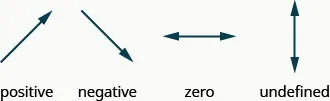Sometimes we’ll need to find the slope of a line between two points when we don’t have a graph to count out the rise and the run. We could plot the points on grid paper, then count out the rise and the run, but as we’ll see, there is a way to find the slope without graphing. Before we get to it, we need to introduce some algebraic notation.

We have seen that an ordered pair $(x,y)(x,y)$ gives the coordinates of a point. But when we work with slopes, we use two points. How can the same symbol $(x,y)(x,y)$ be used to represent two different points? Mathematicians use subscripts to distinguish the points.

$(x1,y1)read “xsub 1,ysub 1” (x2,y2)read “xsub 2,ysub 2” (x1,y1)read “xsub 1,ysub 1” (x2,y2)read “xsub 2,ysub 2”$

We will use $(x1,y1)(x1,y1)$ to identify the first point and $(x2,y2)(x2,y2)$ to identify the second point.

If we had more than two points, we could use $(x3,y3),(x3,y3),$$(x4,y4),(x4,y4),$ and so on.

Let’s see how the rise and run relate to the coordinates of the two points by taking another look at the slope of the line between the points $(2,3)(2,3)$ and $(7,6),(7,6),$ as shown in this graph.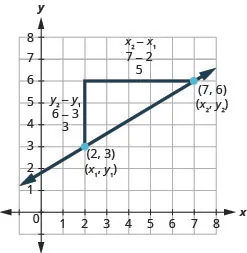Since we have two points, we will use subscript notation. $(2,x1,3y1)(7,6x2,y2)(2,x1,3y1)(7,6x2,y2)$ $m=riserunm=riserun$ On the graph, we counted the rise of 3 and the run of 5. $m=35m=35$ Notice that the rise of 3 can be found by subtracting they-coordinates, 6 and 3, and the run of 5 can be found bysubtracting the x-coordinates 7 and 2. We rewrite the rise and run by putting in the coordinates. $m=6−37−2m=6−37−2$ But 6 is $y2,y2,$ the y-coordinate of the second point and 3 is $y1,y1,$ the y-coordinateof the first point. So we can rewrite the slope using subscript notation. $m=y2−y17−2m=y2−y17−2$ Also 7 is the x-coordinate of the second point and 2 is the x-coordinateof the first point. So again we rewrite the slope using subscript notation. $m=y2−y1x2−x1m=y2−y1x2−x1$

We’ve shown that $m=y2−y1x2−x1m=y2−y1x2−x1$ is really another version of $m=riserun.m=riserun.$ We can use this formula to find the slope of a line when we have two points on the line.

### Slope of a line between two points

The slope of the line between two points $(x1,y1)(x1,y1)$ and $(x2,y2)(x2,y2)$ is:

$m=y2−y1x2−x1.m=y2−y1x2−x1.$

The slope is:

$yof the second point minusyof the first point over xof the second point minusxof the first point. yof the second point minusyof the first point over xof the second point minusxof the first point.$

### Example 3.14

Use the slope formula to find the slope of the line through the points $(−2,−3)(−2,−3)$ and $(−7,4).(−7,4).$

### Try It 3.27

Use the slope formula to find the slope of the line through the pair of points: $(−3,4)(−3,4)$ and $(2,−1).(2,−1).$

### Try It 3.28

Use the slope formula to find the slope of the line through the pair of points: $(−2,6)(−2,6)$ and $(−3,−4).(−3,−4).$

### Graph a Line Given a Point and the Slope

Up to now, in this chapter, we have graphed lines by plotting points, by using intercepts, and by recognizing horizontal and vertical lines.

We can also graph a line when we know one point and the slope of the line. We will start by plotting the point and then use the definition of slope to draw the graph of the line.

### Example 3.15

#### How to graph a Line Given a Point and the Slope

Graph the line passing through the point $(1,−1)(1,−1)$ whose slope is $m=34.m=34.$

### Try It 3.29

Graph the line passing through the point $(2,−2)(2,−2)$ with the slope$m=43.m=43.$

### Try It 3.30

Graph the line passing through the point $(−2,3)(−2,3)$ with the slope $m=14.m=14.$

### How To

#### Graph a line given a point and the slope.

1. Step 1. Plot the given point.
2. Step 2. Use the slope formula $m=riserunm=riserun$ to identify the rise and the run.
3. Step 3. Starting at the given point, count out the rise and run to mark the second point.
4. Step 4. Connect the points with a line.

### Graph a Line Using its Slope and Intercept

We have graphed linear equations by plotting points, using intercepts, recognizing horizontal and vertical lines, and using one point and the slope of the line. Once we see how an equation in slope–intercept form and its graph are related, we’ll have one more method we can use to graph lines.

See Figure 3.6. Let’s look at the graph of the equation $y=12x+3y=12x+3$ and find its slope and y-intercept.

Figure 3.6

The red lines in the graph show us the rise is 1 and the run is 2. Substituting into the slope formula:

$m=riserun m=12 m=riserun m=12$

The y-intercept is $(0,3).(0,3).$

Look at the equation of this line.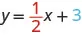Look at the slope and y-intercept.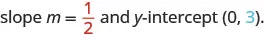When a linear equation is solved for y, the coefficient of the x term is the slope and the constant term is the y-coordinate of the y-intercept. We say that the equation $y=12x+3y=12x+3$ is in slope–intercept form. Sometimes the slope–intercept form is called the “y-form.”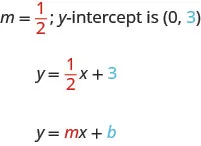### Slope Intercept Form of an Equation of a Line

The slope–intercept form of an equation of a line with slope m and y-intercept, $(0,b)(0,b)$ is $y=mx+b.y=mx+b.$

Let’s practice finding the values of the slope and y-intercept from the equation of a line.

### Example 3.16

Identify the slope and y-intercept of the line from the equation:

$y=−47x−2y=−47x−2$ $x+3y=9x+3y=9$

### Try It 3.31

Identify the slope and y-intercept from the equation of the line.

$y=25x−1y=25x−1$ $x+4y=8x+4y=8$

### Try It 3.32

Identify the slope and y-intercept from the equation of the line.

$y=−43x+1y=−43x+1$ $3x+2y=123x+2y=12$

We have graphed a line using the slope and a point. Now that we know how to find the slope and y-intercept of a line from its equation, we can use the y-intercept as the point, and then count out the slope from there.

### Example 3.17

Graph the line of the equation $y=−x+4y=−x+4$ using its slope and y-intercept.

### Try It 3.33

Graph the line of the equation $y=−x−3y=−x−3$ using its slope and y-intercept.

### Try It 3.34

Graph the line of the equation$y=−x−1y=−x−1$ using its slope and y-intercept.

Now that we have graphed lines by using the slope and y-intercept, let’s summarize all the methods we have used to graph lines.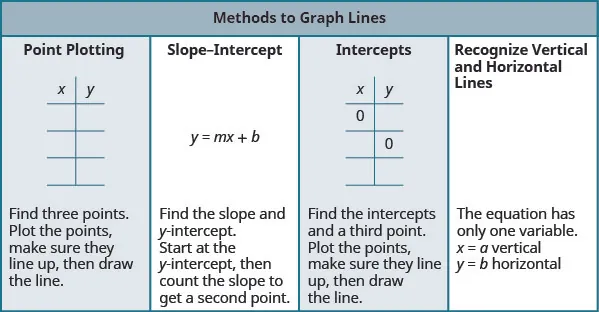### Choose the Most Convenient Method to Graph a Line

Now that we have seen several methods we can use to graph lines, how do we know which method to use for a given equation?

While we could plot points, use the slope–intercept form, or find the intercepts for any equation, if we recognize the most convenient way to graph a certain type of equation, our work will be easier.

Generally, plotting points is not the most efficient way to graph a line. Let’s look for some patterns to help determine the most convenient method to graph a line.

Here are five equations we graphed in this chapter, and the method we used to graph each of them.

$EquationMethod#1x=2Vertical line#2y=−1Horizontal line#3−x+2y=6Intercepts#44x−3y=12Intercepts#5y=−x+4Slope–interceptEquationMethod#1x=2Vertical line#2y=−1Horizontal line#3−x+2y=6Intercepts#44x−3y=12Intercepts#5y=−x+4Slope–intercept$

Equations #1 and #2 each have just one variable. Remember, in equations of this form the value of that one variable is constant; it does not depend on the value of the other variable. Equations of this form have graphs that are vertical or horizontal lines.

In equations #3 and #4, both x and y are on the same side of the equation. These two equations are of the form $Ax+By=C.Ax+By=C.$ We substituted $y=0y=0$ to find the x- intercept and $x=0x=0$ to find the y-intercept, and then found a third point by choosing another value for x or y.

Equation #5 is written in slope–intercept form. After identifying the slope and y-intercept from the equation we used them to graph the line.

This leads to the following strategy.

### Strategy for Choosing the Most Convenient Method to Graph a Line

Consider the form of the equation.

• If it only has one variable, it is a vertical or horizontal line.
• $x=ax=a$ is a vertical line passing through the x-axis at a.
• $y=by=b$ is a horizontal line passing through the y-axis at b.
• If y is isolated on one side of the equation, in the form $y=mx+b,y=mx+b,$ graph by using the slope and y-intercept.
• Identify the slope and y-intercept and then graph.
• If the equation is of the form $Ax+By=C,Ax+By=C,$ find the intercepts.
• Find the x- and y-intercepts, a third point, and then graph.

### Example 3.18

Determine the most convenient method to graph each line:

$y=5y=5$ $4x−5y=204x−5y=20$ $x=−3x=−3$ $y=−59x+8y=−59x+8$

### Try It 3.35

Determine the most convenient method to graph each line:

$3x+2y=123x+2y=12$ $y=4y=4$ $y=15x−4y=15x−4$ $x=−7.x=−7.$

### Try It 3.36

Determine the most convenient method to graph each line:

$x=6x=6$ $y=−34x+1y=−34x+1$ $y=−8y=−8$ $4x−3y=−1.4x−3y=−1.$

### Graph and Interpret Applications of Slope–Intercept

Many real-world applications are modeled by linear equations. We will take a look at a few applications here so you can see how equations written in slope–intercept form relate to real world situations.

Usually, when a linear equation models uses real-world data, different letters are used for the variables, instead of using only x and y. The variable names remind us of what quantities are being measured.

Also, we often will need to extend the axes in our rectangular coordinate system to bigger positive and negative numbers to accommodate the data in the application.

### Example 3.19

The equation $F=95C+32F=95C+32$ is used to convert temperatures, C, on the Celsius scale to temperatures, F, on the Fahrenheit scale.

Find the Fahrenheit temperature for a Celsius temperature of 0.

Find the Fahrenheit temperature for a Celsius temperature of 20.

Interpret the slope and F-intercept of the equation.

Graph the equation.

### Try It 3.37

The equation $h=2s+50h=2s+50$ is used to estimate a woman’s height in inches, h, based on her shoe size, s.

Estimate the height of a child who wears women’s shoe size 0.

Estimate the height of a woman with shoe size 8.

Interpret the slope and h-intercept of the equation.

Graph the equation.

### Try It 3.38

The equation $T=14n+40T=14n+40$ is used to estimate the temperature in degrees Fahrenheit, T, based on the number of cricket chirps, n, in one minute.

Estimate the temperature when there are no chirps.

Estimate the temperature when the number of chirps in one minute is 100.

Interpret the slope and T-intercept of the equation.

Graph the equation.

The cost of running some types of business has two components—a fixed cost and a variable cost. The fixed cost is always the same regardless of how many units are produced. This is the cost of rent, insurance, equipment, advertising, and other items that must be paid regularly. The variable cost depends on the number of units produced. It is for the material and labor needed to produce each item.

### Example 3.20

Sam drives a delivery van. The equation $C=0.5m+60C=0.5m+60$ models the relation between his weekly cost, C, in dollars and the number of miles, m, that he drives.

Find Sam’s cost for a week when he drives 0 miles.

Find the cost for a week when he drives 250 miles.

Interpret the slope and C-intercept of the equation.

Graph the equation.

### Try It 3.39

Stella has a home business selling gourmet pizzas. The equation $C=4p+25C=4p+25$ models the relation between her weekly cost, C, in dollars and the number of pizzas, p, that she sells.

Find Stella’s cost for a week when she sells no pizzas.

Find the cost for a week when she sells 15 pizzas.

Interpret the slope and C-intercept of the equation.

Graph the equation.

### Try It 3.40

Loreen has a calligraphy business. The equation $C=1.8n+35C=1.8n+35$ models the relation between her weekly cost, C, in dollars and the number of wedding invitations, n, that she writes.

Find Loreen’s cost for a week when she writes no invitations.

Find the cost for a week when she writes 75 invitations.

Interpret the slope and C-intercept of the equation.

Graph the equation.

### Use Slopes to Identify Parallel and Perpendicular Lines

Two lines that have the same slope are called parallel lines. Parallel lines have the same steepness and never intersect.

We say this more formally in terms of the rectangular coordinate system. Two lines that have the same slope and different y-intercepts are called parallel lines. See Figure 3.7.

Figure 3.7

Verify that both lines have the same slope, $m=25,m=25,$ and different y-intercepts.

What about vertical lines? The slope of a vertical line is undefined, so vertical lines don’t fit in the definition above. We say that vertical lines that have different x-intercepts are parallel, like the lines shown in this graph.

Figure 3.8

### Parallel Lines

Parallel lines are lines in the same plane that do not intersect.

• Parallel lines have the same slope and different y-intercepts.
• If $m1m1$ and $m2m2$ are the slopes of two parallel lines then $m1=m2.m1=m2.$
• Parallel vertical lines have different x-intercepts.

Since parallel lines have the same slope and different y-intercepts, we can now just look at the slope–intercept form of the equations of lines and decide if the lines are parallel.

### Example 3.21

Use slopes and y-intercepts to determine if the lines are parallel:

$3x−2y=63x−2y=6$ and $y=32x+1y=32x+1$ $y=2x-3y=2x-3$ and $−6x+3y=−9.−6x+3y=−9.$

### Try It 3.41

Use slopes and y-intercepts to determine if the lines are parallel:

$2x+5y=52x+5y=5$ and $y=−25x−4y=−25x−4$ $y=−12x−1y=−12x−1$ and $x+2y=−2.x+2y=−2.$

### Try It 3.42

Use slopes and y-intercepts to determine if the lines are parallel:

$4x−3y=64x−3y=6$ and $y=43x−1y=43x−1$ $y=34x−3y=34x−3$ and $3x−4y=12.3x−4y=12.$

### Example 3.22

Use slopes and y-intercepts to determine if the lines are parallel:

$y=−4y=−4$ and $y=3y=3$ $x=−2x=−2$ and $x=−5.x=−5.$

### Try It 3.43

Use slopes and y-intercepts to determine if the lines are parallel:

$y=8y=8$ and $y=−6y=−6$ $x=1x=1$ and $x=−5.x=−5.$

### Try It 3.44

Use slopes and y-intercepts to determine if the lines are parallel:

$y=1y=1$ and $y=−5y=−5$ $x=8x=8$ and $x=−6.x=−6.$

Let’s look at the lines whose equations are $y=14x−1y=14x−1$ and $y=−4x+2,y=−4x+2,$ shown in Figure 3.9.

Figure 3.9

These lines lie in the same plane and intersect in right angles. We call these lines perpendicular.

If we look at the slope of the first line, $m1=14,m1=14,$ and the slope of the second line, $m2=−4,m2=−4,$ we can see that they are negative reciprocals of each other. If we multiply them, their product is $−1.−1.$

$m1·m214(−4)−1m1·m214(−4)−1$

This is always true for perpendicular lines and leads us to this definition.

### Perpendicular Lines

Perpendicular lines are lines in the same plane that form a right angle.

• If $m1m1$ and $m2m2$ are the slopes of two perpendicular lines, then:
• their slopes are negative reciprocals of each other, $m1=−1m2.m1=−1m2.$
• the product of their slopes is $−1−1$, $m1·m2=−1.m1·m2=−1.$
• A vertical line and a horizontal line are always perpendicular to each other.

We were able to look at the slope–intercept form of linear equations and determine whether or not the lines were parallel. We can do the same thing for perpendicular lines.

We find the slope–intercept form of the equation, and then see if the slopes are opposite reciprocals. If the product of the slopes is $−1,−1,$ the lines are perpendicular.

### Example 3.23

Use slopes to determine if the lines are perpendicular:

$y=−5x−4y=−5x−4$ and $x−5y=5x−5y=5$ $7x+2y=37x+2y=3$ and $2x+7y=52x+7y=5$

### Try It 3.45

Use slopes to determine if the lines are perpendicular:

$y=−3x+2y=−3x+2$ and $x−3y=4x−3y=4$ $5x+4y=15x+4y=1$ and $4x+5y=3.4x+5y=3.$

### Try It 3.46

Use slopes to determine if the lines are perpendicular:

$y=2x−5y=2x−5$ and $x+2y=−6x+2y=−6$ $2x−9y=32x−9y=3$ and $9x−2y=1.9x−2y=1.$

### Section 3.2 Exercises

#### Practice Makes Perfect

Find the Slope of a Line

In the following exercises, find the slope of each line shown.

73.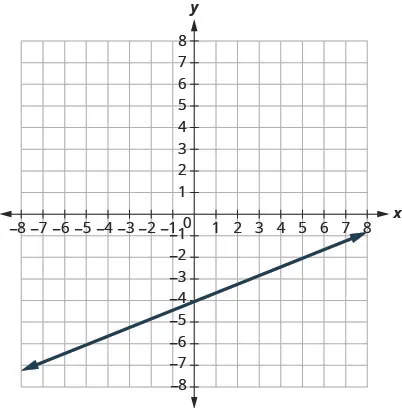74.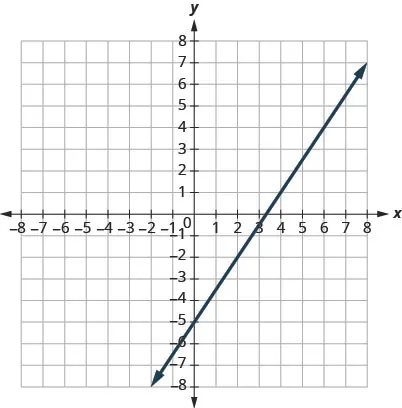75.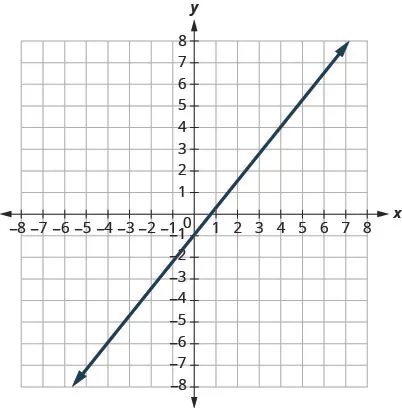76.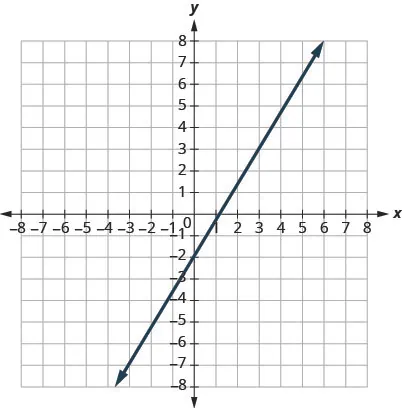77.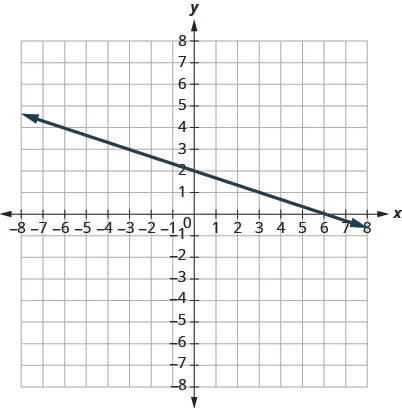78.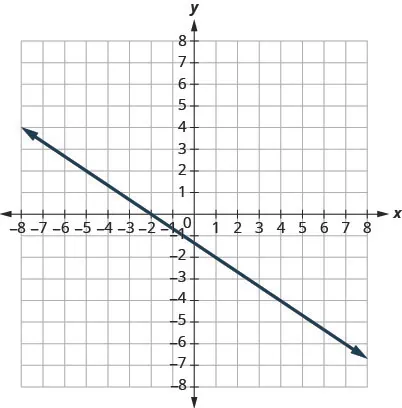79.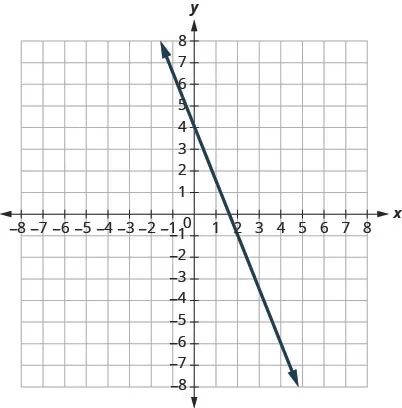80.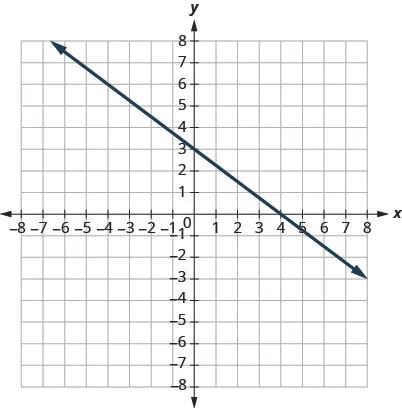In the following exercises, find the slope of each line.

81.

$y = 3 y = 3$

82.

$y = −2 y = −2$

83.

$x = −5 x = −5$

84.

$x = 4 x = 4$

In the following exercises, use the slope formula to find the slope of the line between each pair of points.

85.

$( 2 , 5 ) , ( 4 , 0 ) ( 2 , 5 ) , ( 4 , 0 )$

86.

$( 3 , 6 ) , ( 8 , 0 ) ( 3 , 6 ) , ( 8 , 0 )$

87.

$( −3 , 3 ) , ( 4 , −5 ) ( −3 , 3 ) , ( 4 , −5 )$

88.

$( −2 , 4 ) , ( 3 , −1 ) ( −2 , 4 ) , ( 3 , −1 )$

89.

$( −1 , −2 ) , ( 2 , 5 ) ( −1 , −2 ) , ( 2 , 5 )$

90.

$( −2 , −1 ) , ( 6 , 5 ) ( −2 , −1 ) , ( 6 , 5 )$

91.

$( 4 , −5 ) , ( 1 , −2 ) ( 4 , −5 ) , ( 1 , −2 )$

92.

$( 3 , −6 ) , ( 2 , −2 ) ( 3 , −6 ) , ( 2 , −2 )$

Graph a Line Given a Point and the Slope

In the following exercises, graph each line with the given point and slope.

93.

$( 2 , 5 ) ; ( 2 , 5 ) ;$ $m = − 1 3 m = − 1 3$

94.

$(1,4)(1,4)$; $m=−12m=−12$

95.

$(−1,−4)(−1,−4)$; $m=43m=43$

96.

$(−3,−5)(−3,−5)$; $m=32m=32$

97.

y-intercept 3; $m=−25m=−25$

98.

x-intercept $−2−2$; $m=34m=34$

99.

$(−4,2)(−4,2)$; $m=4m=4$

100.

$(1,5)(1,5)$; $m=−3m=−3$

Graph a Line Using Its Slope and Intercept

In the following exercises, identify the slope and y-intercept of each line.

101.

$y = −7 x + 3 y = −7 x + 3$

102.

$y = 4 x − 10 y = 4 x − 10$

103.

$3 x + y = 5 3 x + y = 5$

104.

$4 x + y = 8 4 x + y = 8$

105.

$6 x + 4 y = 12 6 x + 4 y = 12$

106.

$8 x + 3 y = 12 8 x + 3 y = 12$

107.

$5 x − 2 y = 6 5 x − 2 y = 6$

108.

$7 x − 3 y = 9 7 x − 3 y = 9$

In the following exercises, graph the line of each equation using its slope and y-intercept.

109.

$y = 3 x − 1 y = 3 x − 1$

110.

$y = 2 x − 3 y = 2 x − 3$

111.

$y = − x + 3 y = − x + 3$

112.

$y = − x − 4 y = − x − 4$

113.

$y = − 2 5 x − 3 y = − 2 5 x − 3$

114.

$y = − 3 5 x + 2 y = − 3 5 x + 2$

115.

$3 x − 2 y = 4 3 x − 2 y = 4$

116.

$3 x − 4 y = 8 3 x − 4 y = 8$

Choose the Most Convenient Method to Graph a Line

In the following exercises, determine the most convenient method to graph each line.

117.

$x = 2 x = 2$

118.

$y = 5 y = 5$

119.

$y = −3 x + 4 y = −3 x + 4$

120.

$x − y = 5 x − y = 5$

121.

$x − y = 1 x − y = 1$

122.

$y = 2 3 x − 1 y = 2 3 x − 1$

123.

$3 x − 2 y = −12 3 x − 2 y = −12$

124.

$2 x − 5 y = −10 2 x − 5 y = −10$

Graph and Interpret Applications of Slope–Intercept

125.

The equation $P=31+1.75wP=31+1.75w$ models the relation between the amount of Tuyet’s monthly water bill payment, P, in dollars, and the number of units of water, w, used.

Find Tuyet’s payment for a month when 0 units of water are used.

Find Tuyet’s payment for a month when 12 units of water are used.

Interpret the slope and P-intercept of the equation.

Graph the equation.

126.

The equation $P=28+2.54wP=28+2.54w$ models the relation between the amount of R and y’s monthly water bill payment, P, in dollars, and the number of units of water, w, used.

Find the payment for a month when R and y used 0 units of water.

Find the payment for a month when R and y used 15 units of water.

Interpret the slope and P-intercept of the equation.

Graph the equation.

127.

Bruce drives his car for his job. The equation $R=0.575m+42R=0.575m+42$ models the relation between the amount in dollars, R, that he is reimbursed and the number of miles, m, he drives in one day.

Find the amount Bruce is reimbursed on a day when he drives 0 miles.

Find the amount Bruce is reimbursed on a day when he drives 220 miles.

Interpret the slope and R-intercept of the equation.

Graph the equation.

128.

Janelle is planning to rent a car while on vacation. The equation $C=0.32m+15C=0.32m+15$ models the relation between the cost in dollars, C, per day and the number of miles, m, she drives in one day.

Find the cost if Janelle drives the car 0 miles one day.

Find the cost on a day when Janelle drives the car 400 miles.

Interpret the slope and C-intercept of the equation.

Graph the equation.

129.

Cherie works in retail and her weekly salary includes commission for the amount she sells. The equation $S=400+0.15cS=400+0.15c$ models the relation between her weekly salary, S, in dollars and the amount of her sales, c, in dollars.

Find Cherie’s salary for a week when her sales were $0. Find Cherie’s salary for a week when her sales were$3,600.

Interpret the slope and S-intercept of the equation.

Graph the equation.

130.

Patel’s weekly salary includes a base pay plus commission on his sales. The equation $S=750+0.09cS=750+0.09c$ models the relation between his weekly salary, S, in dollars and the amount of his sales, c, in dollars.

Find Patel’s salary for a week when his sales were 0.

Find Patel’s salary for a week when his sales were 18,540.

Interpret the slope and S-intercept of the equation.

Graph the equation.

131.

Costa is planning a lunch banquet. The equation $C=450+28gC=450+28g$ models the relation between the cost in dollars, C, of the banquet and the number of guests, g.

Find the cost if the number of guests is 40.

Find the cost if the number of guests is 80.

Interpret the slope and C-intercept of the equation.

Graph the equation.

132.

Margie is planning a dinner banquet. The equation $C=750+42gC=750+42g$ models the relation between the cost in dollars, C of the banquet and the number of guests, g.

Find the cost if the number of guests is 50.

Find the cost if the number of guests is 100.

Interpret the slope and C-intercept of the equation.

Graph the equation.

Use Slopes to Identify Parallel and Perpendicular Lines

In the following exercises, use slopes and y-intercepts to determine if the lines are parallel, perpendicular, or neither.

133.

$y = 3 4 x − 3 ; 3 x − 4 y = −2 y = 3 4 x − 3 ; 3 x − 4 y = −2$

134.

$3 x + 4 y = − 2 ; y = 3 4 x − 3 3 x + 4 y = − 2 ; y = 3 4 x − 3$

135.

$2 x − 4 y = 6 ; x − 2 y = 3 2 x − 4 y = 6 ; x − 2 y = 3$

136.

$8 x + 6 y = 6 ; 12 x + 9 y = 12 8 x + 6 y = 6 ; 12 x + 9 y = 12$

137.

$x = 5 ; x = −6 x = 5 ; x = −6$

138.

$x = − 3 ; x = − 2 x = − 3 ; x = − 2$

139.

$4 x − 2 y = 5 ; 3 x + 6 y = 8 4 x − 2 y = 5 ; 3 x + 6 y = 8$

140.

$8 x − 2 y = 7 ; 3 x + 12 y = 9 8 x − 2 y = 7 ; 3 x + 12 y = 9$

141.

$3 x − 6 y = 12 ; 6 x − 3 y = 3 3 x − 6 y = 12 ; 6 x − 3 y = 3$

142.

$9 x − 5 y = 4 ; 5 x + 9 y = − 1 9 x − 5 y = 4 ; 5 x + 9 y = − 1$

143.

$7 x − 4 y = 8 ; 4 x + 7 y = 14 7 x − 4 y = 8 ; 4 x + 7 y = 14$

144.

$5 x − 2 y = 11 ; 5 x − y = 7 5 x − 2 y = 11 ; 5 x − y = 7$

145.

$3 x − 2 y = 8 ; 2 x + 3 y = 6 3 x − 2 y = 8 ; 2 x + 3 y = 6$

146.

$2 x + 3 y = 5 ; 3 x − 2 y = 7 2 x + 3 y = 5 ; 3 x − 2 y = 7$

147.

$3 x − 2 y = 1 ; 2 x − 3 y = 2 3 x − 2 y = 1 ; 2 x − 3 y = 2$

148.

$2 x + 4 y = 3 ; 6 x + 3 y = 2 2 x + 4 y = 3 ; 6 x + 3 y = 2$

149.

$y = 2 ; y = 6 y = 2 ; y = 6$

150.

$y = − 1 ; y = 2 y = − 1 ; y = 2$

#### Writing Exercises

151.

How does the graph of a line with slope $m=-12m=-12$ differ from the graph of a line with slope $m=2?m=2?$

152.

Why is the slope of a vertical line “undefined”?

153.

Explain how you can graph a line given a point and its slope.

154.

Explain in your own words how to decide which method to use to graph a line.

#### Self Check

After completing the exercises, use this checklist to evaluate your mastery of the objectives of this section.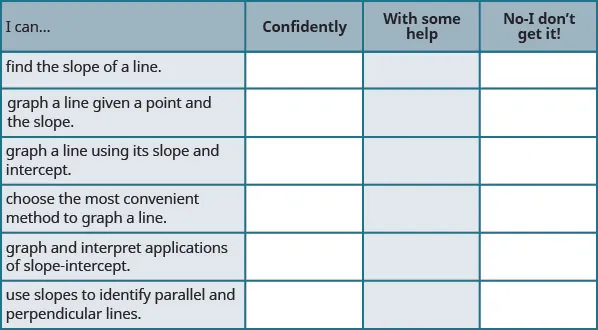After reviewing this checklist, what will you do to become confident for all objectives?

Order a print copy

As an Amazon Associate we earn from qualifying purchases.